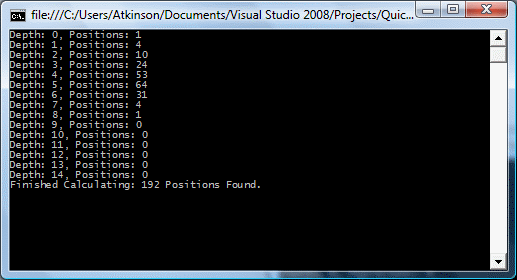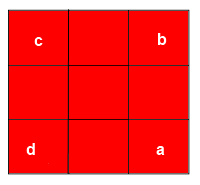# Visual C# GuideFloppy Cube Project - Back To The Problem

If you built the program as described, you should get the following output at the console,Assuming that the numbers are correct, we can interpret the results to mean that there are 192 unique positions for the puzzle and that a maximum of 8 turns is required to solve the puzzle from any position. We also find that there is only one position that requires 8 turns.

## Are We Correct?

Before we started, we could have done maths to consider what our maximum number of positions would be.

With 4 corner pieces that can occupy 4 corners positions, we have 4! permutations of corners. That is 4x3x2x1 = 24.

With 4 edge pieces, each having one of two possible orientations, we have 24 ways that the edges could be flipped. That is, 2x2x2x2 = 16.

Multiply these two figures together and there are 24 x 16 = 384 conceivable ways in which the edges and corners might be arranged.

That appears to be exactly double the figure that we have found. Are we wrong?

These numbers work if the cubies aren't connected to some sort of mechanism as they are with this puzzle. But these cubies are. When dealing with puzzles, the number of swaps or flips matters. When you swap a pair of corners, you also flip an edge. A running total of the number of corner swaps would always be equal to the running total of the number of edge swaps. This means that there is a limit to the way our cubies can actually be arranged on the puzzle.

You can tell that some of the arrangements can't be recreated on the puzzle by trying to swap two diagonally opposite corner pieces. It takes 3 turns to do this - can't be done quicker. This means 3 corner-swaps. It also means flipping 3 edges. An odd number of edge flips must leave at least one edge flipped. The following position is not therefore possible,Half of the corner positions will require an odd number of swaps to reach, edge positions needing an even number of swaps to reach are discounted. The odd numbered positons are discounted for the other half of the positions. The total number of positions can be divided by 2 leaving us with the 192 that our program found.

The problem was as follows,

• How many unique states can the puzzle be placed in using only combinations of the 4 turns (without rotating the whole puzzle)?
• What is the maximum number of turns required to solve the puzzle?
• What are the combinations of turns required to solve the puzzle from each of these positions?

The answer to the first question we now know is 192. We can also see from our table that the maximum number of turns required to solve the puzzle (its diameter) is 8.

For the last question, we will need to output our sequences and test them out on a puzzle,

The following procedure shows how to access all of the items in the dictionary using a foreach.

```private static void outputMoveTable() {    foreach(KeyValuePair<string,string> kvp in moveTable)    {       Console.WriteLine("{0}: {1}", kvp.Key, kvp.Value);    } }```

The first thing to check, if you have a puzzle to hand, is that the moves generate the postions described. Go with the ones which are simple to check first. Next scramble the puzzle for as long as you can, without remembeing what you did. Work out the cube state and search for it. Do the reverse of the sequence of moves and see if you have the solved puzzle.